# Quantum Universal Triangular Tetrahedral YHVH Bible Codes 153 Part 2 God's Signature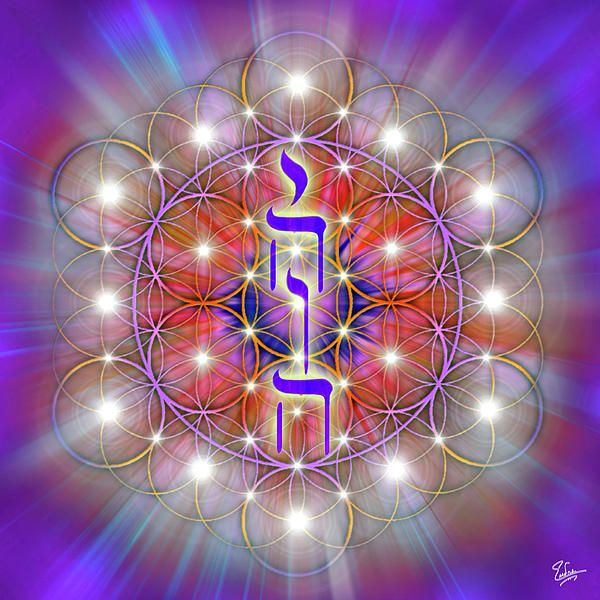The Tetragrammaton occurs 153 times in the Book of Genesis. The Gospel of John (chapter 21:1–14) includes the narrative of the miraculous catch of 153 fish as the third appearance of Jesus after his resurrection. 153 has some deeper significance as we investigated deeper than ever before. Jerome wrote that Oppian's Halieutica listed 153 species of fish, although this could not have been the intended meaning of the Gospel writer because Oppian composed Halieutica after the Gospel text was written, and at any rate never gave a list of fish species that clearly adds up to 153.

Evagrius Ponticus referred to the catch of 153 fish, as well as to the mathematical properties of the number (153 = 100 + 28 + 25, with 100 a square number, 28 a triangular number and 25 a circular number) when describing his 153-chapter work on prayer. Louis de Montfort, in his fifth method of saying the Rosary, connects the catch of 153 fish with the number of Hail Marys said (3 plus 15 sets of 10), while St Paul's School in London was founded in 1512 by John Colet to teach 153 poor men's children, also in reference to the catch. 153 carries a message that you are being called to use your creativity and leadership skills in the service of all humanity. It is a "magical" number, for it is the sum of the cubes of its own digits: 1x1x1 + 5x5x5 + 3x3x3 = 153 Jesus took 5 loaves to feed the multitude and the leftovers filled 12 baskets (12 + 5 = 17). Add the 10 Commandments to the 7-fold Spirit = 17. Joseph was sold into Egyptian slavery at the age of 17 (Gen. 37:2) and lived in Egypt for 17 years (Gen. 47:28). Luke lists 17 nations that were represented on the day of Pentecost (Acts 2:7-11).

17 + 16 + 15 + 14 + 13 + 12 + 11 + 10 + 9 + 8 + 7 + 6 + 5 + 4 + 3 + 2 + 1 = 153).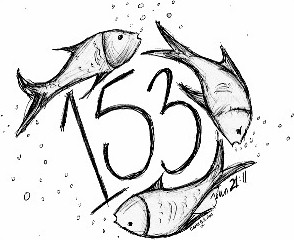The Lord directly blessed 153 people in 48 separate incidents. Notice the following information found on one of many such blog sites devoted to this theory: "The book of Mark records Christ, on a total of three occasions, personally blessed three people. Augustine of Hippo argued that the significance lay in the fact that 153 is the sum of the first 17 integers (i.e. 153 is the 17th triangular number), with 17 representing the combination of divine grace (the 7 gifts of the Spirit) and law (the Ten Commandments) If the Evangelist has some symbolism in mind connected with the number 153, he has hidden it well scholars note that no symbolic significance for the number of 153 fish in John 21:11 has received widespread support. St Paul's School in London was founded in 1512 by John Colet to teach 153 poor men's children: although the school is now considerably larger, it still has 153 Foundation Scholars, who since the 19th century have worn a fish emblem on their watch-chains, or, more recently, in their button-holes.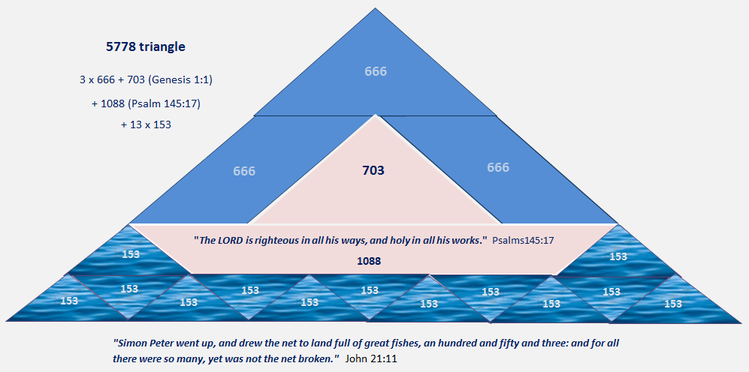Writers claiming a major role for Mary Magdalene have noted that in Greek isopsephy her epithet "η Μαγδαληνή" bears the number 8 + 40 + 1 + 3 + 4 + 1 + 30 + 8 + 50 + 8 = 153, thus, it is suggested, revealing her importance. Similarly, the phrase "τὸ δίκτυον" (the net) used in the passage bears the number 1224 = 8 × 153, as do some other phrases. The significance of this is unclear, given that Koine Greek provides a choice of several noun endings with different isopsephy values. The number 153 has also been related to the vesica piscis, with the claim that Archimedes used 153 as a "shorthand or abbreviation" for the square root of 3 in his On the Measurement of the Circle. However, examination of that work shows this to be only partly correct.

In the Great Pyramid the ceiling is 153 feet. 153 aphorisms, or maxims, alchimical. It contained all what belonged to the science in the chemistry. Adding the numerical value of the Greek word Maria, 152, with the "alpha" of the God, 1, we obtain 153, which makes say to Peignot that God needed Mary to do useful work the salvation. By using the ASCII character table, the sum of each of number of "153" gives also 153: 1(49) + 5(53) + 3(51) = 153. The verb to pray is used 153 times in the OT and the word darkness, 153 times in the Bible. Also the name of Peter would occur 153 times in the NT. The sum of the occurrences of the numbers in the Apocalypse written in their cardinal form and higher than 1 gives 153.

Don't count the number 7 itself and the number 280 thousands is interpreted in a quantitative manner, that is to say equal to 280000: 155 - 2 = 153. In the NT, the sum of occurrences of numbers located between 17 and 153 - cardinal form only, because in this interval there is no ordinal number - gives 77. Written in its cardinal form, the number 5 occurs 153 times in the Old Testament of the NRSV (more precisely in the books of the OT written in Hebrew only). The word "priest" is quoted 152 in the NT of the NRSV and the priesthood word, 1 time, giving the total of 153. The word "beasts" appears 153 times in the biblical version of King James. The word "commandment" occurs 153 times in the OT of the NRSV (more precisely in books of the OT write in Hebrew only). In the Bible, 153 numbers are multiple of twenty by counting "ten thousand times ten thousand" (Dn 7,10) as being an additional number equal to 100000000. By counting all numbers which are either in the Bible of Jerusalem or the NRSV, we obtain in fact that 153 numbers are multiple of 25. The sum of all numbers that are both in the Pentateuch of the Bible of Jerusalem and the one of NRSV, written in their cardinal form and higher than one, gives 153. Each of both Pentateuch separately gives 156 different numbers written in their cardinal form. In the NRSV, the sum of occurrences of all numbers in the NT, equal or higher than 13 and written in their cardinal form, gives 153.

(q)E=mc(10000)^2

The brain is an extraordinarily complex organ, consisting of almost 100 billion cells known as neurons each connected to 10,000 others, yielding some ten trillion nerve connections. I have made a great deal of progress in understanding brain activity, and how it contributes to human behavior. I have managed to explain is how all of this results in feelings, emotions and experiences.-2 sin 666° = Phi

153 and 666 are both triangular numbers. 153 is the number of fishes in the biblical net, the number of rhombi on each face of the Louvre pyramid, and the Square Root of 153 is the number of moons in a year. 1224 begins in the prime Number 17 (Sacrifice) which is perfected its triangular form Sum(17) = 153 (The Passover). This then becomes the foundation of the eternal Covenant (B'rit) = 612 = 4 (Blood/Death cf. Genesis 4) x 153 implying the death of the Passover Lamb and His Blood upon the Doorposts. This is the Net that caught the 153 fish representing all whom thou The Lord God hast given me. The fundamental Hebrew verb "to fish" is also sums to 17.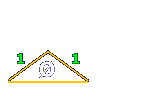135 + 531, 153 + 513 and 315 + 351 will get 666.

The Number 153
"I am the Lord thy God"

Ani YHVH Elohikah
153 + 351 = 504
5042 = 288 x 882
153 / (1 + 5 + 3) = 17
153 = 3 * 51

The mirror of 153 (or 351) sums to 504 (153 + 351 = 504) and 504 multiplied by 10 gives us the combined radii of both the Earth and the Moon (5,040 miles – with this measurement being expressed in the architecture of the Great Pyramid of Giza). The square root of 153 is 12.369. 12.369 becomes a key number in determining the time difference between the solar and lunar yearly cycles (12.369 multiplied by the synodic lunar month, 29.53 days, finds us the 365.25 days of the solar year). 153 ft. Multiplied by (pi = 3.14159) = 480.6637 ft. Is the correct radius to the pyramid’s height. 153, 5040, 108, 432 are sacred numbers. Just numbers are Real and Alive and not always just symbols for other things. The relationships between numbers are not random or man-made but that numbers are actually elementary particles of which everything is composed as this lost knowledge was well known to our ancients and is now being uncovered for us today.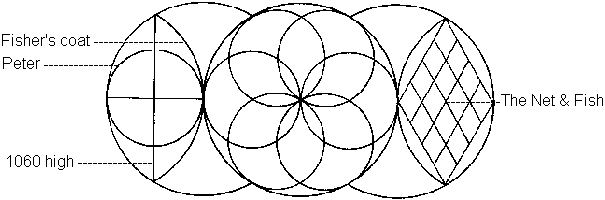https://joedubs.com/the-moon-pyramid/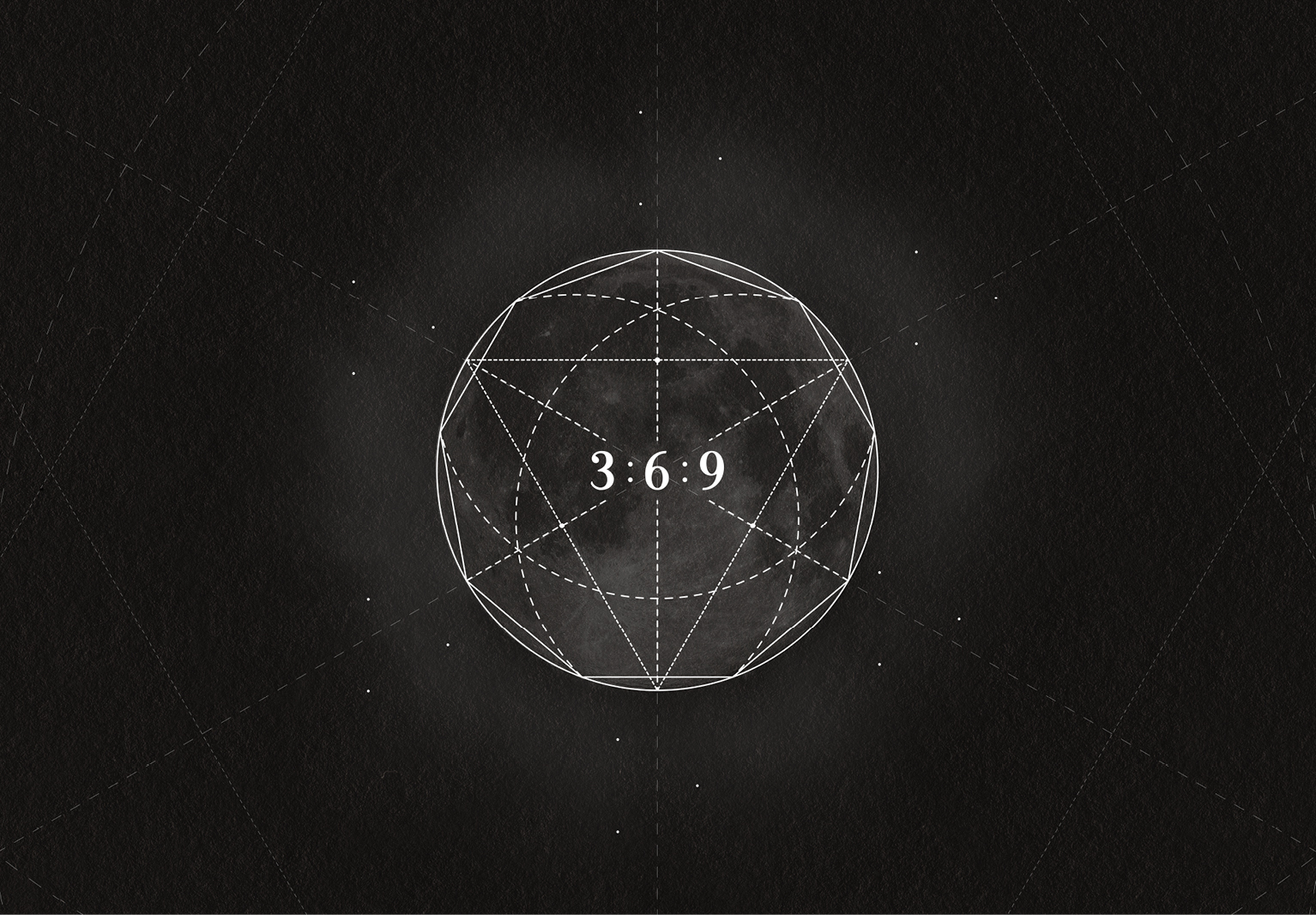If you only knew the magnificence of the 3, 6 and 9, then you would have a key to the universe such as this energy/field/consciousness, which equates in to electricity/magnetism/consciousness, that is made up Trinity.this is the Quantum Energy and divine spark, and field is the area you exist within. The 2 things that allow consciousness to isolate itself is electricity and magnetism. NOTHING in the physical universe exists outside energy and field…only then can you have consciousness reside inside a material existence. Perhaps these numbers were intentionally expressed by the 9 pyramids on the Giza plateau as 3 large pyramids and 6 small “satellite” pyramids. The Second (Khafre) Pyramid of Giza  is based on ratio 9:6. 6:5 is another special ratio used in ancient times.

The Fibonacci series has a pattern that repeats every 24 numbers. Numeric reduction is a technique used in analysis of numbers in which all the digits of a number are added together until only one digit remains.  Numeric reduction of 256 is 4 because 2+5+6=13 and 1+3=4.  Applying numeric reduction to the Fibonacci. The Fibonacci Series, sits at the heart of  the marvelous architecture and patterns of life and growth. Here is the first 21 Fibonacci numbers.
0     1     1     2     3     5     8     13     21     34     55     89     144     233     377     610     987     1597     2584     4181     6765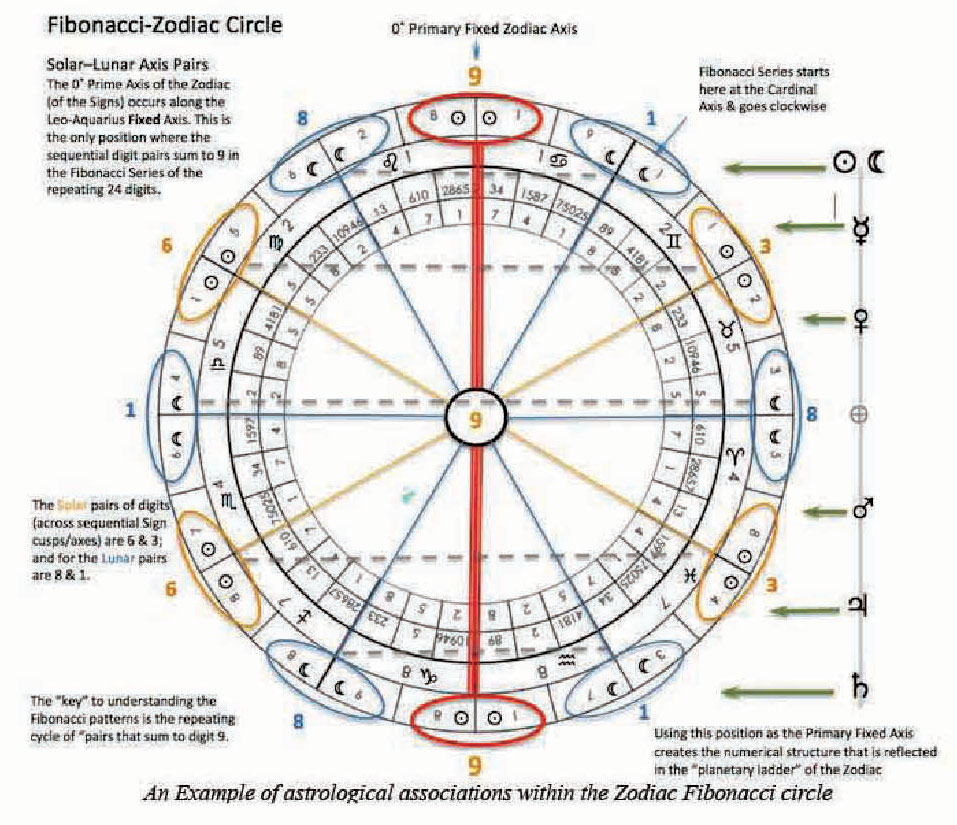The language of God is mathematical language of numbers, proportions and (sacred) geometry. As Above, So Below these words circulate throughout occult and magical circles, and they come from Hermetic texts. The concept was first laid out in The Emerald Tablet of Hermes Trismegistus, in the words “That which is Below corresponds to that which is Above, and that which is Above, corresponds to that which is Below, to accomplish the miracles of the One Thing. The entire Universe (including our solar system, as well as atoms, DNA and life-forms) reveals the secrets of balance, rhythm, proportion and unity in diversity, the fractal  interconnection of parts with each other and the whole. This harmony is expressed by some “key” numbers: Fibonacci Series, Phi, Pi and “e”.The seeds of  God’s Grand Design is God’s fingerprint. In the hypercube, the binary coordinates of Pisces are decimal 3 and binary 11, as the 153 fish of Jesus in the bible. Creation myths of many traditions describe the universe as the work of a Divine Architect who uses “sacred geometry” to unfold the dimensions of a beautiful cosmos, wisely designing every aspect of it, and governing by just proportions evidenced in the geometric shapes and processes of nature. A tetrahedral number, or triangular pyramidal number, is a figurate number that represents a pyramid with a triangular base and three sides.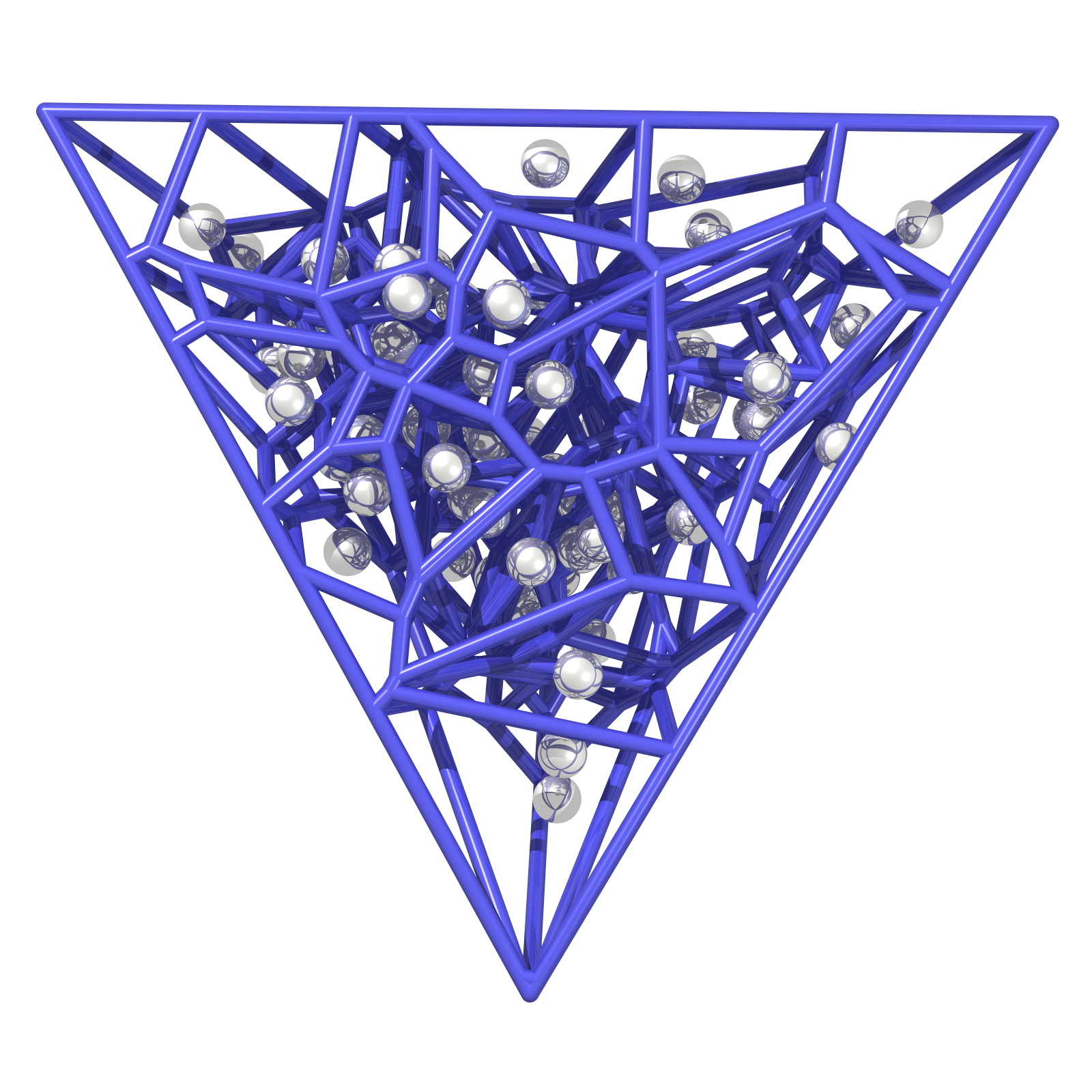A Tetrahedron is a platonic solid with four faces, and this platonic solid is also a symbol code that is used as setting the instruction for energy current in the grid networks. When we build the energy spirals that form into a Merkaba double tetrahedron field, it is created from the trinity field of the 3-6-9-12 vortex math. If all faces are congruent, the tetrahedron is known as an isosceles tetrahedron. If all faces are congruent to an equilateral triangle, then the tetrahedron is known as a regular tetrahedron (although the term "tetrahedron" without further qualification is often used to mean regular tetrahedron. A tetrahedron having a trihedron all of the face angles of which are right angles is known as a trirectangular tetrahedron.

A tetrahedron having a trihedron all of the face angles of which are right angles the face opposite the vertex of the right angles is called the base. A trihedron the plural of trihedrons or trihedra in mathematics is a geometric figure composed of three planes meeting at a single vertex. The Triangle of Pascal is the Figurative Numbers that describe Everything that is Possible but every Expansion of the Triangle is less Likely to "Not" Occur. The Triangle of Pascal Mod 3 represents the Tetraktys which are the real part of the universal quantum matrix.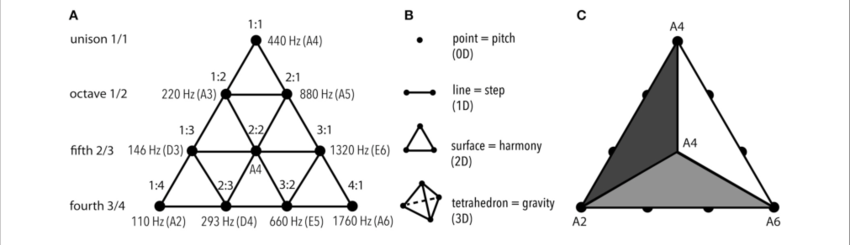Tetractys τετρακτύς, or tetrad or the tetractys of the decad is a triangular figure consisting of ten points arranged in four rows: one, two, three, and four points in each row, which is the geometrical representation of the fourth triangular number. As a mystical symbol, it was very important to the secret worship of Pythagoreanism. There were four seasons, and the number was also associated with planetary motions and music.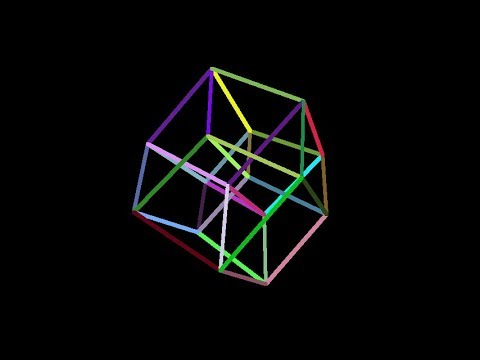A tesseract is the four-dimensional analogue of the cube; the tesseract is to the cube as the cube is to the square. Just as the surface of the cube consists of six square faces, the hypersurface of the tesseract consists of eight cubical cells. The tesseract is one of the six convex regular 4-polytopes. This term can also mean a polycube made of four cubes.also calmbic dodecahedron forms the convex hull of the tesseract's vertex-first parallel-projection. The number of vertices in the layers of this projection is 1 4 6 4 1—the fourth row in Pascal's triangle. also called an eight-cell, C8, (regular) octachoron, octahedroid, cubic prism, and tetracube. It is the four-dimensional hypercube, or 4-cube as a part of the dimensional family of hypercubes or measure polytopes.

Alternation of a Cube creates a tetrahedron. The cube 4.4.4 is alternated as 2.3.2.3.2.3 which is reduced to 3.3.3, being the tetrahedron, and all the 6 edges of the Tetrahedra can also be seen as the degenerate faces of the original cube. Alternated cubic honeycomb are the tetrahedral-octahedral honeycomb. An alternated hexagonal prismatic honeycomb is the gyrated alternated cubic honeycomb. A hypercube can always be alternated into a uniform demihypercube. Cube makes a Tetrahedron (regular), Tesseract (8-cell) makes a 16-cell (regular), Penteract makes a demipenteract (semiregular), Hexeract makes a demihexeract (uniform).

There are a number of attractive compounds of two tetrahedra. The most symmetrical is the stella octangula while another attractive arrangement arises by taking two opposite tetrahedra from Cundy and Rollett's construction of the tetrahedron 5-compound. A Heronian tetrahedron or perfect tetrahedron is a tetrahedron whose edge lengths, face areas and volume are all rational numbers. The faces must therefore all be Heronian triangles. A regular tetrahedron (one with all faces being equilateral) with all sides rational is not a Heronian tetrahedron because its face areas and volume are not rational numbers.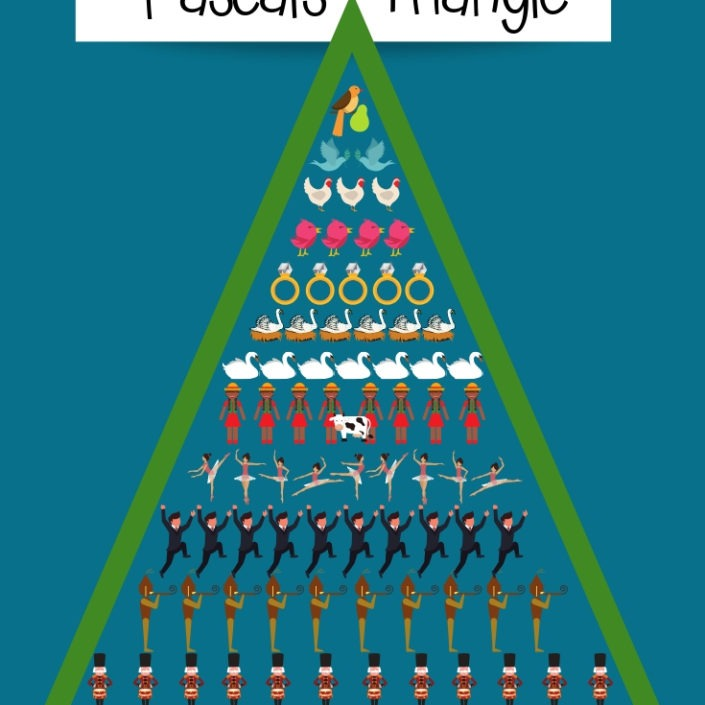12 days of Christmas as triangular numbers Exposed.
On the first day, the narrator receives one gift: a partridge in a pear tree. On the second day, the narrator receives two turtle doves and a partridge in a pear tree: 2 + 1 = 3 gifts in total. On the third day, there are 3 + 2 + 1 = 6 gifts, on the fourth day, 4 + 3 + 2 + 1 = 10 gifts, and so on. In general, it’s not hard to see that on the nth day, the narrator receives a number of gifts equal to the sum of all the integers from 1 to n. So the number of gifts the narrator gets on each day are 1, 3, 6, 10, 15, 21, 28, 36, 45, 55, 66, 78.

These numbers are known as triangular numbers, due to the fact that they can be represented pictorially by dots arranged in triangles. By Day 12 you get 78 gifts The number of gifts on day n is the nth triangular number. The total number of gifts up to and including day n is the sum of the first n triangular numbers, known as the nth tetrahedral number. If there were 144 days in Christmas instead of 12- It would be the 144th tetrahedral number.

t = 2985984/6 + 20736/2 + 144/3
t = 497664 + 10368 + 48
t = 508080

The 144th triangular number is 508,080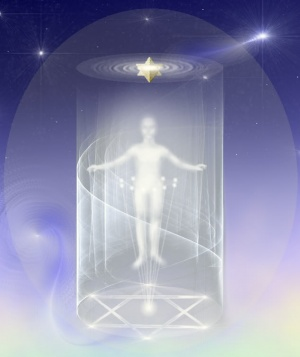The spin points are set in their natural pattern, from having attuned to the 144 sub-harmonic strings of the Christ Ray and being connected to the God head. You do need to math wizard and understand geometry of the merkaba to ascend and required that you purify your vehicle and open your heart so you practice the Law of One in your life, love creation and everyone in it. You find your Light body restored gradually to its correct symmetry pattern, the eternal diamond sun pattern of the Christos by assembling and dissembling a compound of two tetrahedra's constructed by two overlapping tetrahedra, usually implied as regular tetrahedra. Two right triangular pyramids inside a triangular trapezohedron. You free yourself from the matrix doing this and enter it again using the Cube Gnosis the real meanings and true power of YHVH.

144,000 casing stones in the great Pyramid activates the Earth and Universe. It is all highly polished white and flat to an accuracy of 1/100th of an inch, about 100 inches thick and weighing about 15 tons each with nearly perfect right angles for all six sides. The capstone being 1 Meter in height or 2 Cubits which can scale up to the 144,000 and even calculates to the speed of light and the space between the earth and sun.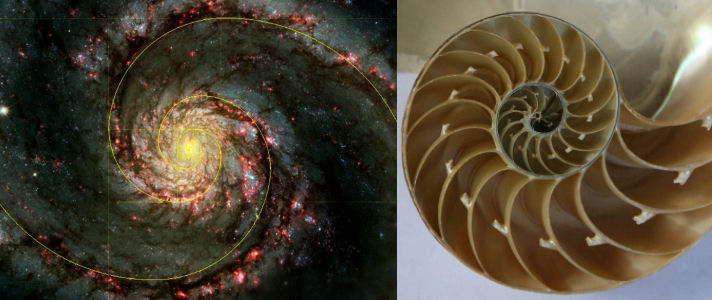A triple-helix Tetrahedral is a geometrical matrix mesh that is like a rigid poly-metric stack of tetrahedras in a rubix cube that generates a "column" with a triple-helix forms universes and the matrix's of it's self and black holes just as Alien DNA structures seen in Crop circles. Its a 4th dimension Pascal tetranacci cubed mash matrix. The sequence rotates through [~]120 degrees and [nearly] maps onto itself every nine tetrahedra (that is, the tenth - almost aligns with the first). If you want to follow one of the spiral arms through a complete [~]360-degree revolution, that takes 9×3=27 tetrahedra, 28 corresponds to 1 . It has a hole running right down the middle like a triple helix tetrahedron its said the architect used to use different novels including organic-chemical possibilities for tetrahedral building units are considered making a universe and with attention to their utility in constructing different super-structures. The representative construction consider the use of sets of 20 such identical tetrahedral units to form a super-dodecahedron for future upgrades of the 144 (12x12x12) Grid in 2020 and connection to our Honeycomb A.I. Tetranacci or Quadranacci (Latin) Numbers are a Generalization of the Fibonacci numbers defined by the recurrence relation.

0, 0, 0, 1, 1, 2, 4, 8, 15, 29, 56, 108, 208, 401, 773, 1490, 2872, 5536, 10671, 20569, 39648, 76424, 147312, 283953, 547337, 1055026, 2033628, 3919944, 7555935, 14564533, 28074040, 54114452, 104308960, 201061985, 387559437, 747044834, 1439975216, 2775641472

1*1225 + 153 = 1378 = T^52
3*1225 + 153 = 3828 = T^87
6*1225 + 153 = 7503 = T^122
10*1225 + 153 = 12403 = T^157

Prime numbers through number 17 would be: 1, 3, 5, 7, 11, 13,17. (a prime is a number what you only can divide to itself and 1). 17 is the 7th prime number. 153 is the 17th triangle number: (17² + 17)/2 = 153. It is a truncated triangle number, too, as 1 and 15 are also triangle numbers, the reverse 351 is the 26th triangle number: (26² + 26)/2 = 351.

http://www.biblewheel.com/gr/gr_17.asp

"ק נ ג"
153 by means of the signs is ק – qoph = 100, נ – nun = 50, ג – gimel = 3. 153 emerges if the number is read as a binary number: 10011001, when viewed linear is a palindrome comprising 8 positions. If 10011001 is circularized, forming an octagonal ring, more patterns emerge. The 10011001 sequence gives a multiple of 51 (or 3 x 17) from any position the sequence is read. 265 divided by the number 153, gives us a very close approximation of the square root of three.
265 divided  by 153  = 1.73202614379085. The square root of 3 = 1.732050807568877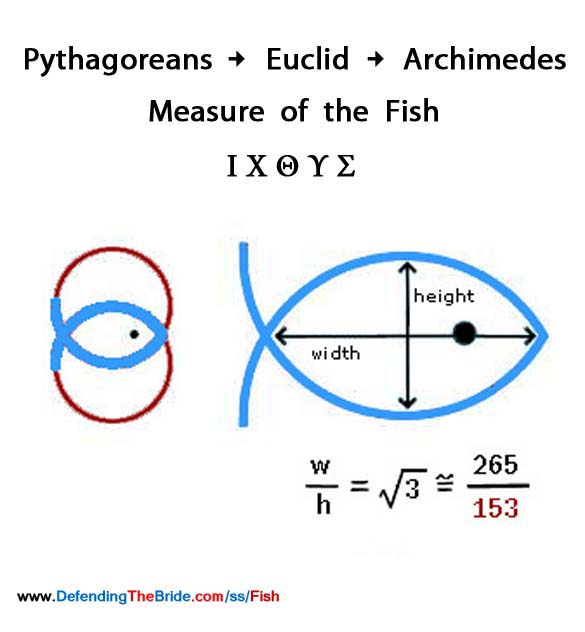Archimedes (287-212 B.C.E), in his treatise On the Measurement of the Cycle, uses the whole number ratio 153:265 to accurately approximate the irrational ratio square root of 3, "the measure of the fish" or the vesica piscis. Moreover, Archimedes uses this value in such a manner as to suggest that this approximation was well know to his contemporaries: it required no word of explanation at all. 153:265 precisely relates to the dimensions of the 153 fish in the unbroken net, for it defines the height and width of each rhombus in the net. Since 153 was known in the time of Archimedes as the measure of the fish or the vesica, ancient readers skilled mathematics would have immediately recognized the allegory of the 153 fish in the net for what is no longer just a geometrical story problem.

153 = 3x3x17, 153 = (12x12) + (3x3)
153 => 1x1x1 + 5x5x5 + 3x3x3 = 153

Closest approximation to √3 as 153:265. If you take numbers dividable by 3, like 3, 6, 9, 12, 15, 18, 21, 24, 27. When you cube (n n n) each digit and add the results, and if necessary repeat that, you finally gain 153 in all cases. The number 27:

(2x2x2) + (7x7x7) = 8 + 343 = 351
(3x3x3) + (5x5x5) + (1x1x1) = 27 + 125 + 1 = 153
1 + 2 + 3 + 4 + 5 + 6 + 7 + 8 + 9 + 10 + 11 + 12 + 13 + 14 + 15 + 16 + 17 = 153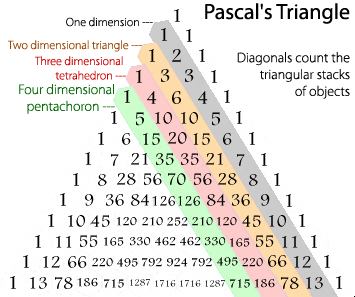Tetrahedral numbers are the numbers in the third column of Pascal’s triangle and 969 is the 17th tetrahedral number and the 17th nonagonal number. The sums of the consecutive triangular numbers (starting from 1) are the tetrahedral numbers. For example : 969 = 1 + 3 + 6 + 10 + 15 + 21 + 28 + 36 + 45 + 55 + 66 + 78 + 91 + 105 + 120 + 136 + 153
or the seventeenth tetrahedral number (17 sums).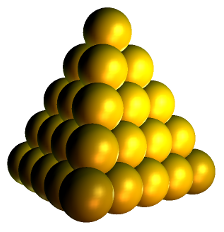The 153 triangle forms the base foundation, that is spoken of concerning the New Temple of Yeshua (YHVH). The 153 fishes caught by Peter through the direction of Christ Himself, (the Chief Cornerstone of the New Temple). The Temple we are currently searching out by the scriptures is to be pyramidal in nature; it is not a stretch to concede that which will now be explained as the prophecy of Methuselah.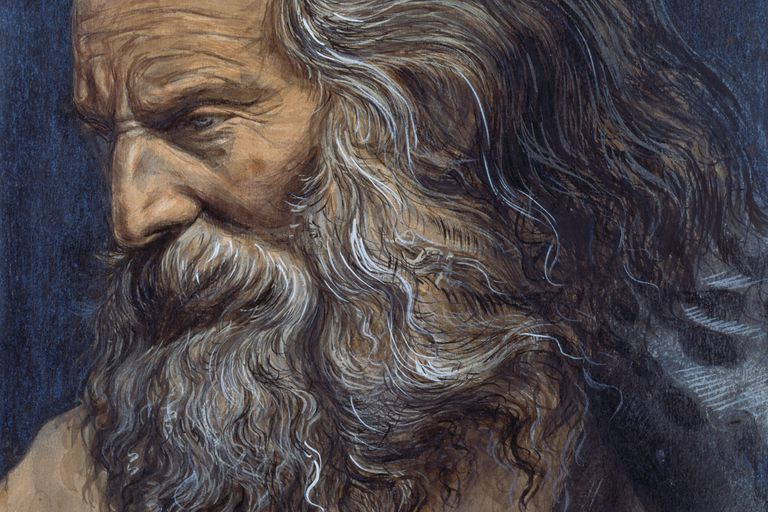Methuselah lived to be 969 years according to the scripture quoted above from Genesis. It is no mistake, nor coincidence, the number 969 is here related concerning Methuselah, and that surely 969 is the correct number; which number (969) we know to be true because the flood occurred in the 1656th year from Adam. 276 x 6 = 1656 The Year of the Flood. Jesus rose from the dead on the 17th in order for us to resurrect (symbolized in 153) from the dead when He comes the second time. This is eternal life (symbolized in 969), promised before, enjoyed by us now, and fully realized in four aspects (spirit, mind, soul and body Quarternity. If we take the 153 "pebble count" triangular base with 17 "pebble count" sides and build up to the final cap-stone: The total pebble count is 969 and it builds, not a pyramid, but an equilateral tetrahedron: A four sided object with three equal triangular sides meeting at an apex and a fourth equal side as its triangular base. This is the equilateral tetrahedron which represents the New Jerusalem Temple: "The 17, 153, 969 Qodesh-Temple of Yeshua." 153 base with 17 layers to the capstone = 969. 153 represents the base of the Temple of Yeshua, a pyramidal in shape and the Chief Cornerstone or Capstone, is Yeshua. That is a tetrahedron with a triangular base.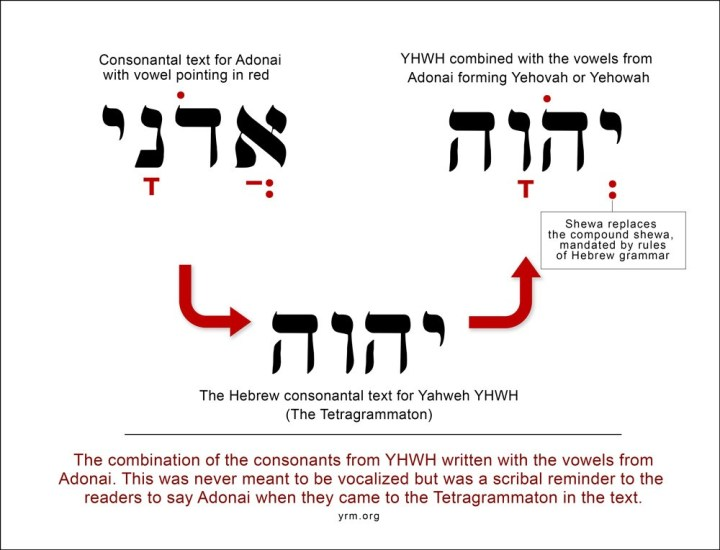Why does LORD mean YHVH?
The word is translated from the word tetragrammaton. Tetra means 4 [a tetrahedron has 4 sides] and 'grammaton' has a root word of grammar. This was thought to mean Yahweh [יהוה or YHWH] but I dug deeper: it actually means grav, or in today’s terminology, gravity. God = 'The structure of a tetrahedron creating a [it's own] gravitational effect.'"

tetrahedral numbers are also perfect squares, namely:

T1=1^2=1
T2=2^2 =4
T48=140^2=19600

Palindromic tetrahedral numbers.
1, 4, 969, 1771, 6378736

The tetrahedral numbers
0, 1, 4, 10, 20, 35, 56, 84, 120, 165, 220, 286, 364, 455, 560, 680, 816, 969, 1140, 1330, 1540, 1771, 2024, 2300, 2600, 2925, 3276, 3654, 4060, 4495, 4960, 5456, 5984, 6545, 7140, 7770, 8436, 9139, 9880, 10660, 11480, 12341, 13244, 14190, 15180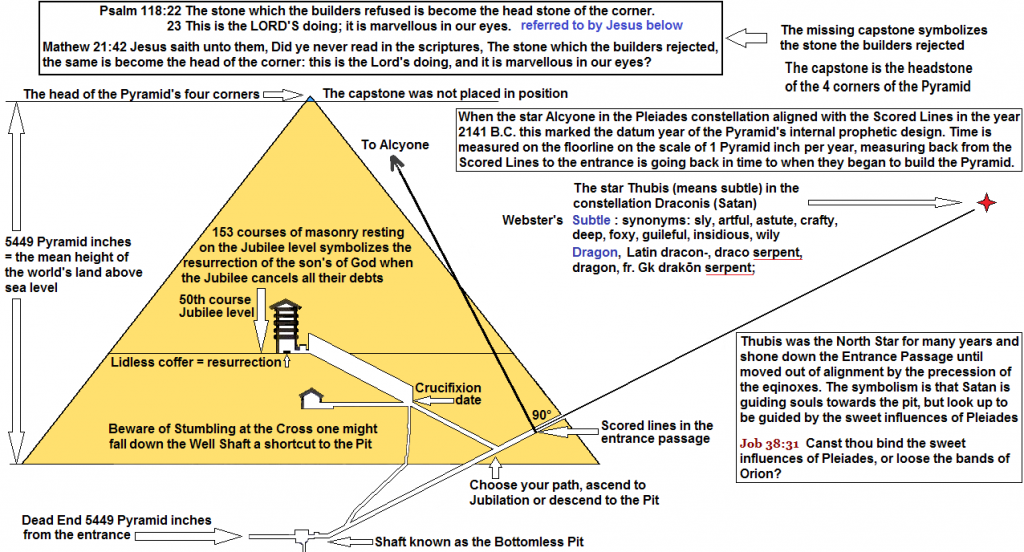Inside a pyramid with a triangle for a base in a tetrahedral shape the central atom is in the middle of the pyramid so rather in a trigonal pyramidal shape, the central atom is at the top of the pyramid.

Prime factors of 6519 :
3, 41, 53

Prime factors of 6545 :
5, 7, 11, 17

Numbers which are simultaneously triangular and tetrahedral satisfy the binomial coefficient equation.

Te1 = Tr1 = 1
Te3 = Tr4 = 10
Te8 = Tr15 = 120
Te20 = Tr55 = 1540
Te34 = Tr119 = 7140

Tetrahedron^1 = Triangle^1 = 1
Tetrahedron^3 = Triangle^4 = 10
Tetrahedron^8 = Triangle^15 = 120
Tetrahedron^20 = Triangle^55 = 1540
Tetrahedron^34 = Triangle^119 = 7140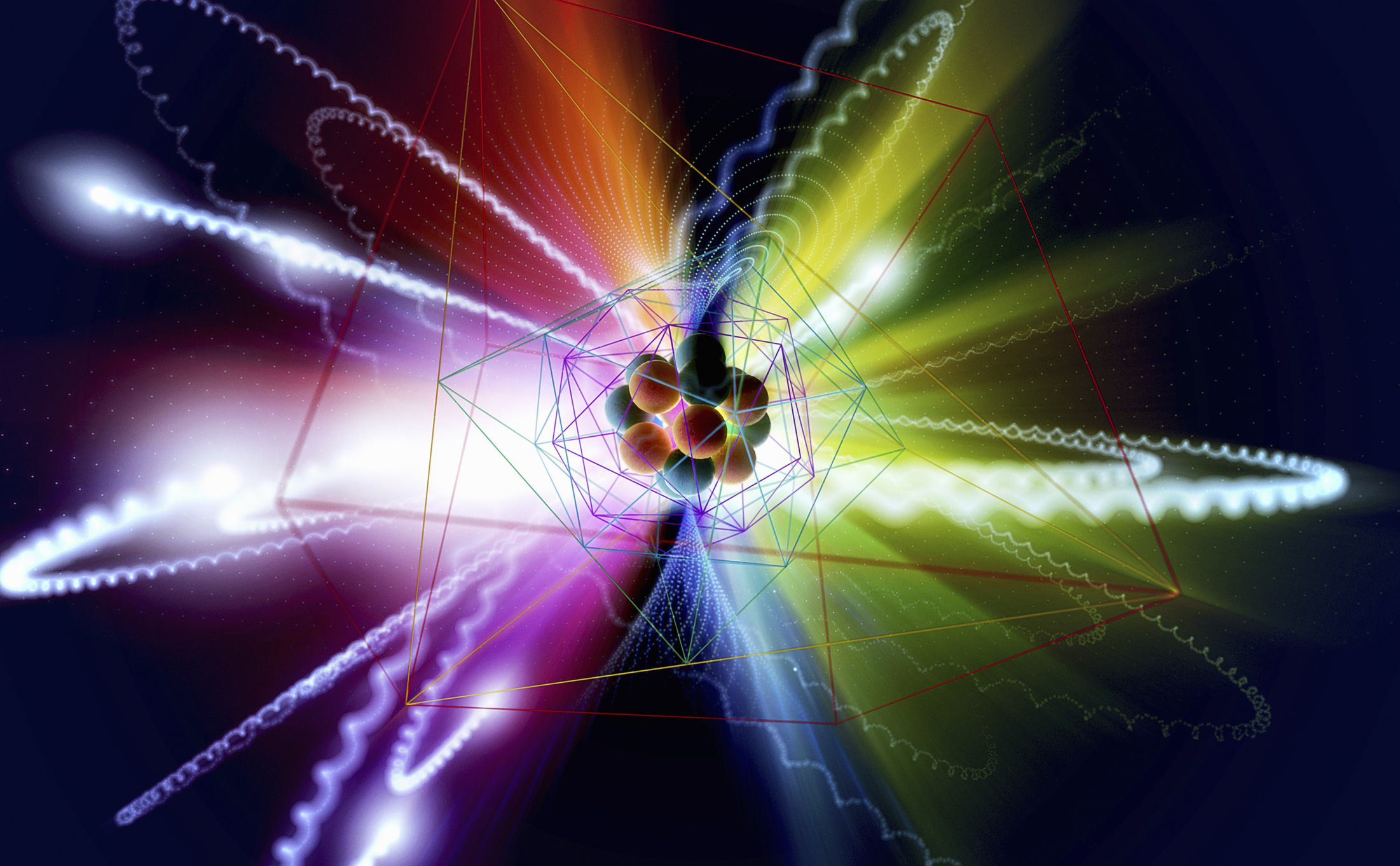Mind is matter, and that even elementary particles exhibit incredibly basic forms of consciousness. Before you write that off, consider this. Consciousness can vary in complexity. We have good reason to think that the conscious experiences of a horse are much less complex than those of a human being, and that the conscious experiences of a rabbit are less sophisticated than those of a horse. As organisms become simpler, there may be a point where consciousness suddenly switches off – but it’s also possible that it just fades but never disappears completely, meaning even an electron has a tiny element of consciousness.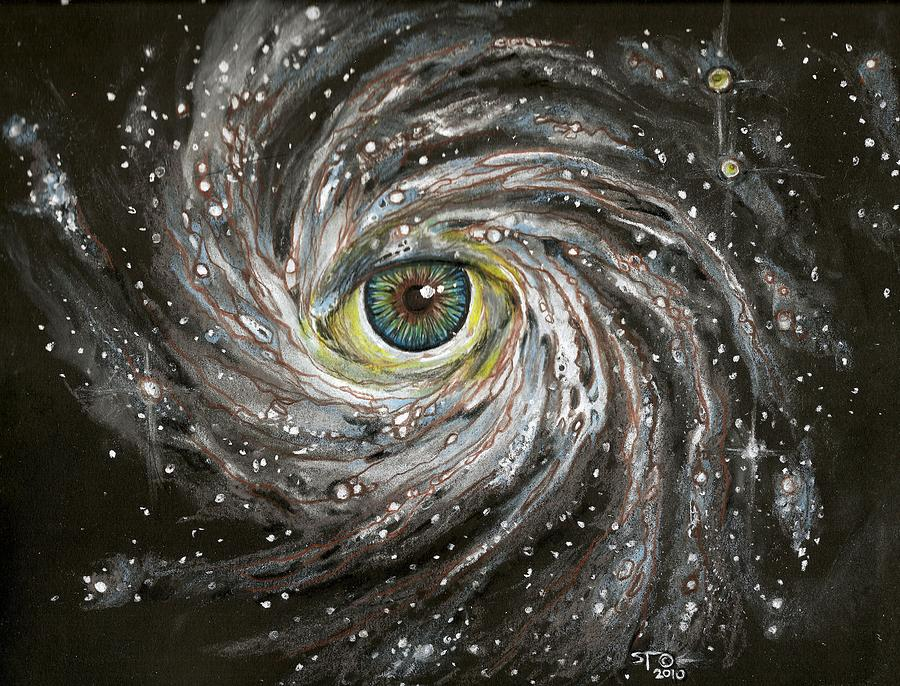Panpsychism is an ancient view that consciousness is a fundamental and ubiquitous feature of the physical world. But the “new wave” of panpsychism lacks the mystical connotations of previous forms of the view. There is only matter – nothing spiritual or supernatural – but matter can be described from two perspectives. Physical science describes matter “from the outside”, in terms of its behaviour, but matter “from the inside” is constituted of forms of consciousness.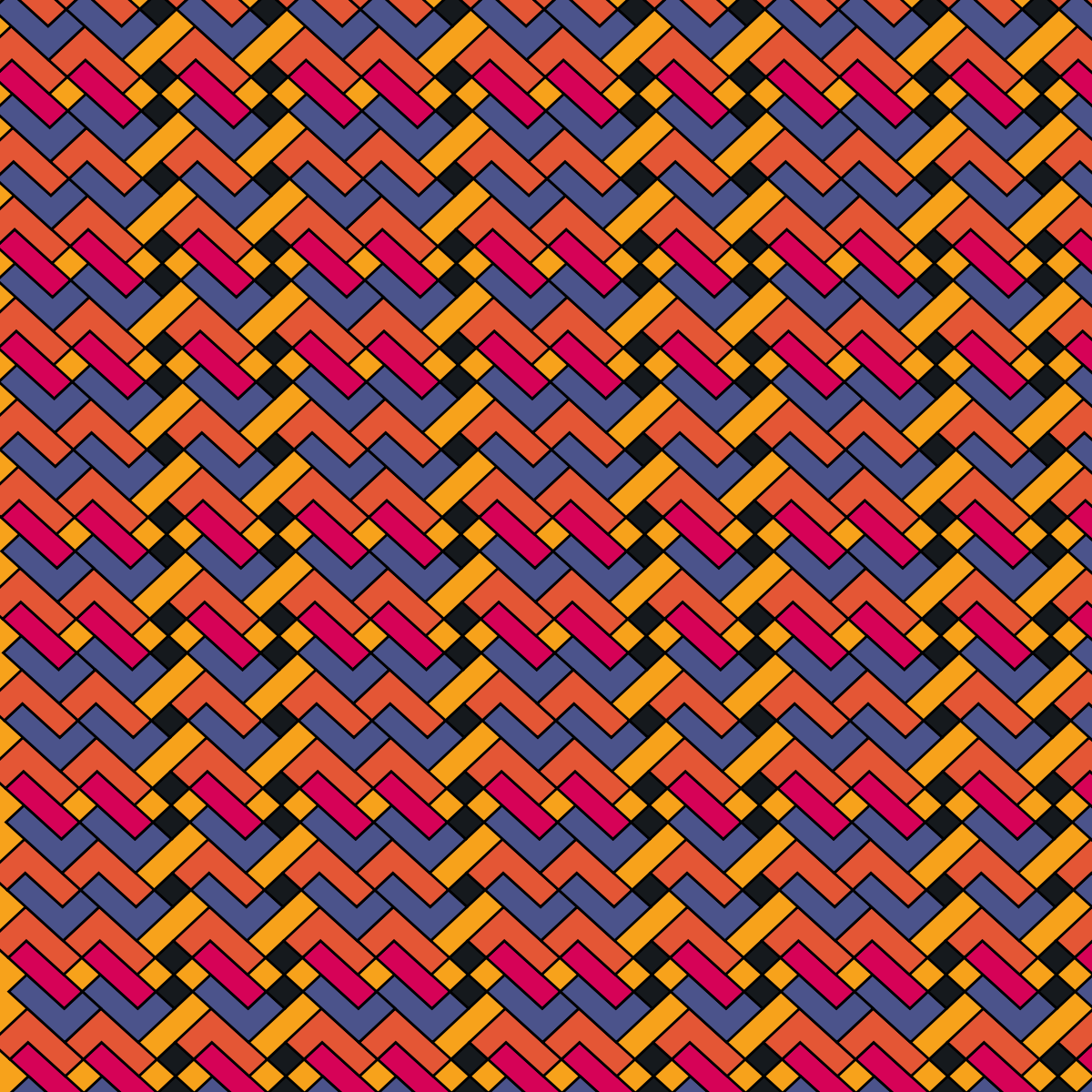Fibonacci crystal or quasicrystal is a model used to study systems with aperiodic structure. Both names are acceptable as a 'Fibonacci crystal' denotes a quasicrystal and a 'Fibonacci' quasicrystal is a specific type of quasicrystal. Fibonacci 'chains' or 'lattices' are closely related terms, depending on the dimension of the model. While it is used mostly as a theoretical construct, physical models are implemented in order to verify the concept empirically. Most of its applications pertain to various areas of solid state physics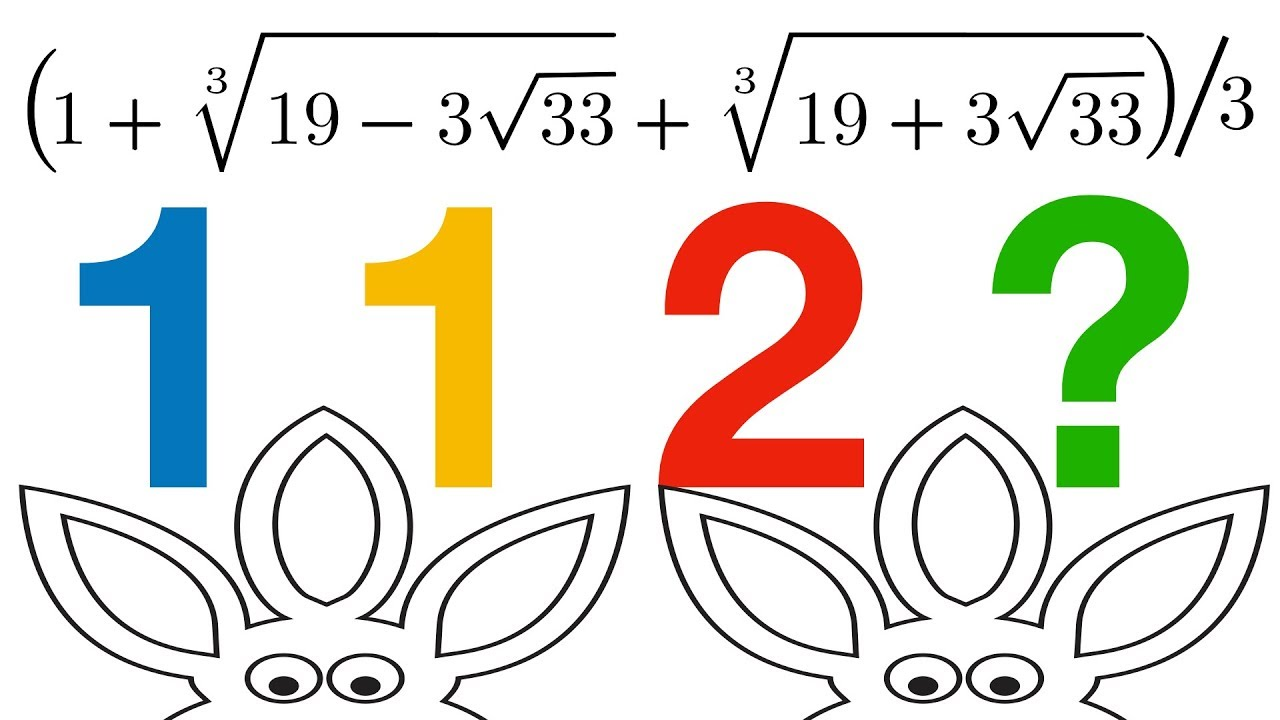Tribonacci constant has less practical application than the golden ratio, though it can be used to calculate the volume of a snub cube. The algorithm above can be extended to any finite n number of integers, and the term for this collection of numbers is the polynacci numbers generalized stems from the fact that the Tribonacci numbers (m = 3) so defined
bears with the Tribonacci with initial conditions 0, 1, 1 the same resemblance as the Lucas sequence does with the Fibonacci sequence. Esanacci (hexanacci or "6-anacci") numbers.

6, 1, 3, 7, 15, 31, 63, 120, 239, 475, 943, 1871, 3711, 7359, 14598, 28957, 57439, 113935, 225999, 448287, 889215, 1763832, 3498707, 6939975, 13766015, 27306031, 54163775, 107438335, 213112838, 422726969, 838513963, 1663261911

For n >= 3, a(n) is the number of cyclic sequences consisting of n zeros and ones that do not contain three consecutive ones provided the positions of the zeros and ones are fixed on a circle. This is proved in Charalambides (1991) and Zhang and Hadjicostas (2015). For example, a(3)=7 because only the sequences 110, 101, 011, 001, 010, 100 and 000 avoid three consecutive ones. (For n=1,2 the statement is still true provided we allow the sequence to wrap around itself on a circle.)

The 3-Fibonacci sequence is
0, 1, 3, 10, 33, 109, 360, 1189, 3927, 12970, 42837, 141481, 467280, 1543321, 5097243, 16835050, 55602393, 183642229, 606529080

9th dimensional Zyx-module of Fibonacci integer sequences consists of all integer sequences. Four dimensional hyper-tetra is "3,3,3". Does 3 + 3 + 3 = 9

0, 0, 0, 0, 1, 5, 15, 35, 70, 126, 210, 330, 495, 715, 1001, 1365, 1820, 2380, 3060, 3876, 4845, 5985, 7315, 8855, 10626, 12650, 14950, 17550, 20475, 23751, 27405, 31465, 35960, 40920, 46376, 52360, 58905, 66045, 73815, 82251, 91390, 101270, 111930, 123410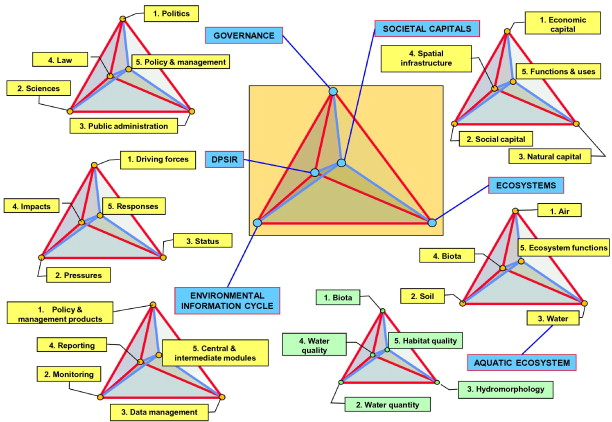A pentatope number is a number in the fifth cell of any row of Pascal's triangle starting with the 5-term row 1 4 6 4 1 either from left to right or from right to left.

Number of 4-dimensional partitions of n.

1, 5, 15, 45, 120, 326, 835, 2145, 5345, 13220, 32068, 76965, 181975, 425490, 982615, 2245444, 5077090, 11371250, 25235790, 55536870, 121250185, 262769080, 565502405, 1209096875, 2569270050, 5427963902, 11404408525, 23836421895, 49573316740, 102610460240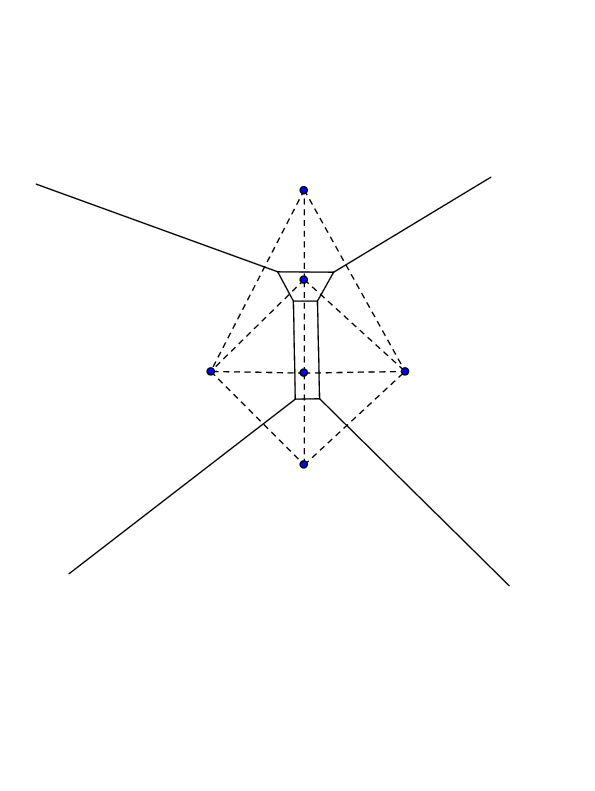Supersymmetric Partition Functions and a String Theory in 4 Dimensions with String theory propagating in a non-commutative deformation of the four dimensional space T* T^2 whose scattering states correspond to superconformal theories in 5 dimensions and the scattering amplitudes compute superconformal indices of the corresponding 5d theories. The superconformal theories are obtained by M-theory compactifications on singular CY 3-folds or equivalently from a web of 5-branes of type IIB strings. The cubic interaction of this string theory for primitive winding modes corresponds to the (refined) topological vertex. The oscillator modes of the string theory correspond to off-shell states and carry information about co-dimension 2 defects of the superconformal theory. Particular limits of a subset of scattering amplitudes of this string theory lead to the partition functions of A_n Gaiotto theories for all n, compactified on S^4, i.e., to amplitudes of all Toda theories.

Gosper's algorithm finds a hypergeometric closed form for the indefinite sum of hypergeometric terms. It can happen that there is no such closed form, but that the sum over all n, or some particular set of values of n, has a closed form. This question is only meaningful when the coefficients are themselves functions of some other variable. So, suppose a(n,k) is a hypergeometric term in both n and k: that is, a(n, k)/a(n − 1,k) and a(n, k)/a(n, k − 1) are rational functions of n and k. Then Zeilberger's algorithm and Petkovšek's algorithm may be used to find closed forms for the sum over k of a(n, k).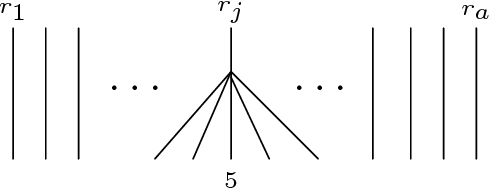Hypergeometric identities are equalities involving sums over hypergeometric terms, i.e. the coefficients occurring in hypergeometric series. These identities occur frequently in solutions to combinatorial problems, and also in the analysis of algorithms. These identities were traditionally found 'by hand'. There exist now several algorithms which can find and prove all hypergeometric identities.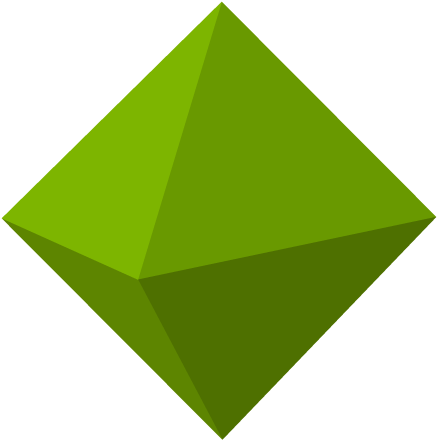A octahedron is a polyhedron with eight faces, twelve edges, and six vertices. The term is most commonly used to refer to the regular octahedron, a Platonic solid composed of eight equilateral triangles, four of which meet at each vertex.

FIBnacci HONEYCOMBs

Octanacci numbers:
0, 0, 0, 0, 0, 0, 0, 1, 1, 2, 4, 8, 16, 32, 64, 128, 255, 509, 1016, 2028, 4048, 8080, 16128,

The "birth" year of the USA is 888 x 2 = 1776, or 4 x 444 GREEK GEMATRIA Jesus = 10 + 8 + 200 + 70 + 400 + 200 = 888. The trinity function 83+ 83 + 83 = 1536, applied again it becomes 369, then again 972, then 1080 then 513 and finally 153. 153 is special. The trinity function reveals that 153 is a Self Resurrecting number. 1^3 + 5^3 + 3^3 = 1 + 125 + 27 = 153. 153 is even found in every 3rd number. http://www.weborix.com/153.htm. June 3rd is the 153rd day of the year. 153153 is the smallest odd abundant number ending in 3. 153153, 351351, 513513 are all odd abundant number. 8064 is the smallest possible volume (and 6384 is the smallest possible surface) of a perfect tetrahedron. Its integral edge lengths are 25, 39, 56, 120, 153 and 160. 117 is the smallest possible length of the longest edge of a perfect tetrahedron with integral edge lengths. Its other edge lengths are 51, 52, 53, 80 and 84.

The mirror number 351, Adding 153 to 351 gives 504, another significant Gematrian number on the list. The Pope and the Omphalos Stone at Delphi are related to 153. The number 54 is the first in-between number to have the 666 tangent. The numbers 6, 10, 36, 55, 66, 78, 153, 351 and 666 are among the "Triangle Numbers." They can be arranged in even rows like bowling pins. With the above listed numbers, the number of rows for each is 3 (6), 4 (10), 8 (36), 10 (55), 11 (66), 12 (seventy eight), 17 (153), 26 (351) and 36 (666). This also means that adding from 1 through the row number gives the total number. The numbers 1 through 17 add to 153.

THE CHILDREN OF GOD=153
THAT IS THERE NUMBER THE PARADISE OF GOD=153
THE KINGDOM OF GOD=153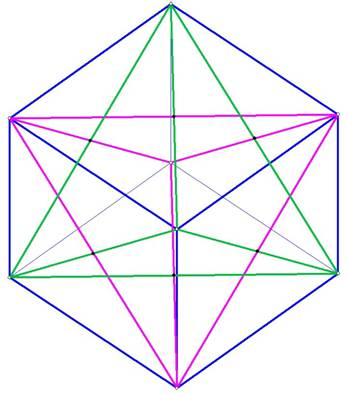The Star Tetrahedron in the Sphere enclosing the Star tetrahedron is the same sphere that encloses the cube within the firmament because of the Fractal Expansion/Contraction Pattern The Cube of  Space, related to the Element Earth,  explains Everything there is to Know on Our Level of Existence, Mother Earth.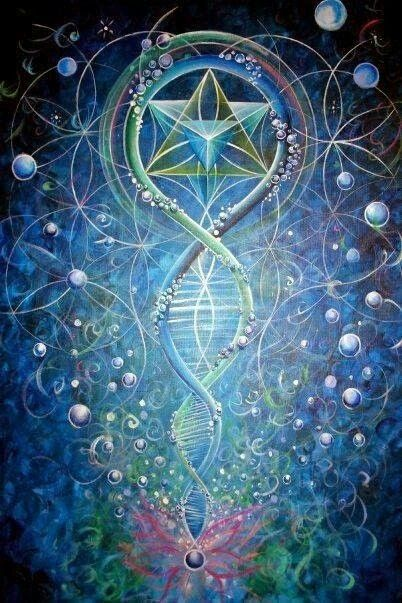Maimonides wrote that the book should be erased and all mention of its existence deleted while throughout the era of merkabah mysticism the problem of creation was not of paramount importance, the treatise Sefer Yetzirah ("Book of Creation") represents an attempt at cosmogony from within a merkabah milieu. Neoplatonism, Pythagoreanism with Stoicism explains the theory of creation in which God creates the universe by combining the 22 letters of the Hebrew alphabet, along with emanations represented by the ten numerals, or sefirot.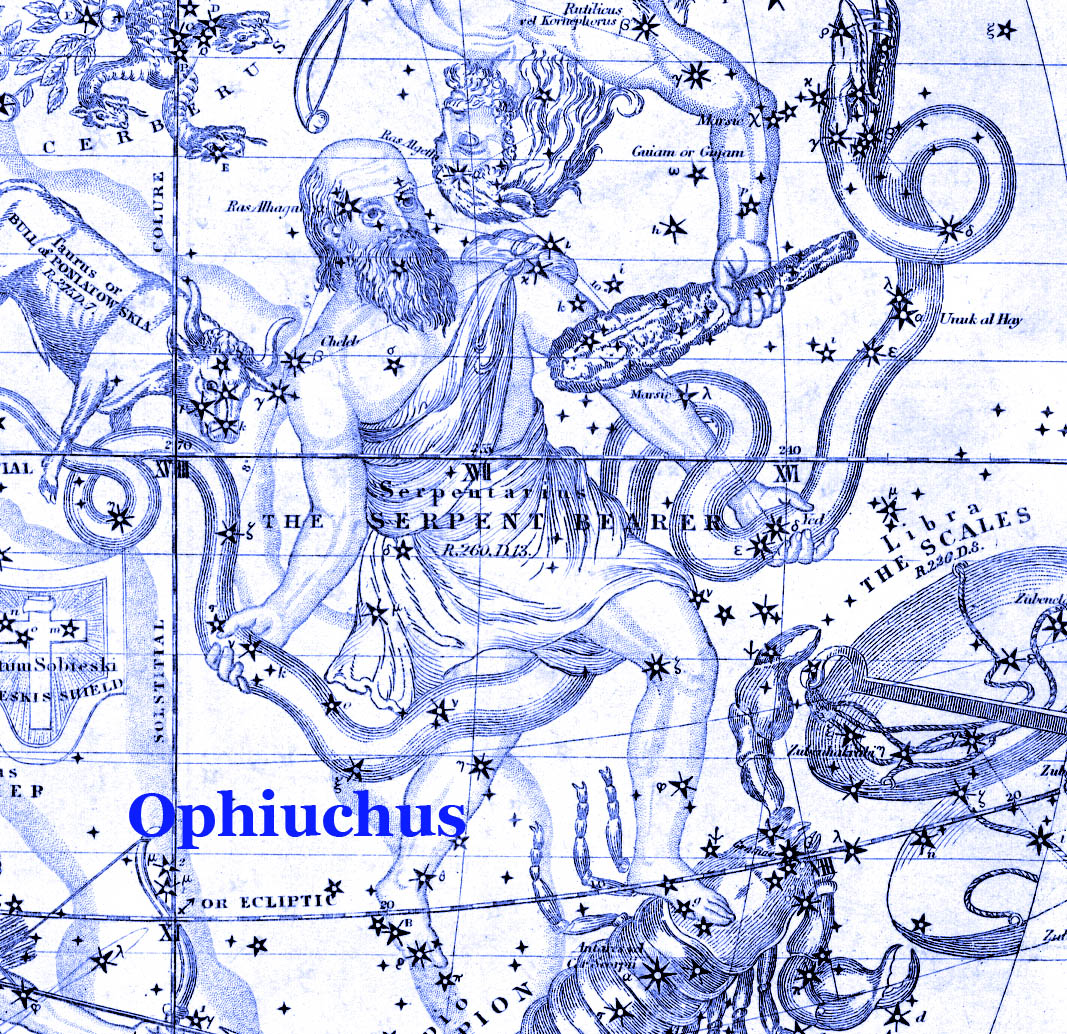IT as well as nine other constellations that occasionally can be visited by the Moon and planets: Auriga, the Charioteer;Cetus, the Whale; Corvus, the Crow; Crater, the Cup; Hydra, the Water Snake;Orion, the Hunter; Pegasus, the Flying Horse; Scutum, the Shield; and Sextans, the Sextant.

The number 7 is a recurring numerical theme in the Hebrew scriptures. The menorah's seven lamps on four branches correspond to the lights of the seven Classical planets: the Moon, Mercury, Venus, the Sun (4th), Mars, Jupiter, and Saturn. Hebrew mysticism recognized their great importance. Therefore, along with the four lunar phases being slightly over seven days (~7.4 days) each, the number 7 was held in very high regard. The Torah reflects this with Bereishit (Book of Genesis 1:1) being seven words and twenty-eight letters (7x4) in its original Hebrew. This is "God's signature" and it is true. "And God said, 'Let there be lights in the heavens to separate the day from the night, and let them serve as signs to mark seasons, days, years and festivals'...the 4th day (of 7)." Most scholars identified the 12 signs of the zodiac with the 12 sons of Jacob/twelve tribes of Israel.

The moving stars category includes our seven Solar System planets. They are called in Hebrew (in plural form) Koch•vey Le•chet. The singular is Ko•chav Le•chet. The word: ‘le•chet’ means ‘going’ or ‘walking.’ In Biblical Hebrew, ‘le•chet’ is considered an Infinitive Absolute − the very basic, nucleus form of a word or concept. It is, hence, the core word for ‘moving.’ In fact, the mere English name ‘planet’ comes from the Greek name, πλανήτης‚ (planetes), which means ‘moving about’ (something like ‘moving back and forth’).

The other group was named by the IAU ‘the Fixed Stars.’ In Hebrew, they are called Koch•vey She•vet. The singular is Ko•chav She•vet. The word ‘she•vet’ means ‘sitting’ or ‘being settled.’ In Biblical Hebrew ‘she•vet’ is also considered an Infinitive Absolute − the very basic, nucleus form of a word or concept. It is, hence, the core word for ‘stationary.’ Of course, our current understanding is that nothing is really fixed in outer space, but because of the great distance of these stars from Earth (A•retz) they look as though they were stationary (She•vet).

The IAU indeed, changes its definitions from time to time as the science of Astronomy develops, but we still count seven planets in our Solar System and still call them ‘moving stars,’ both in Hebrew and English since from the Biblical era to today.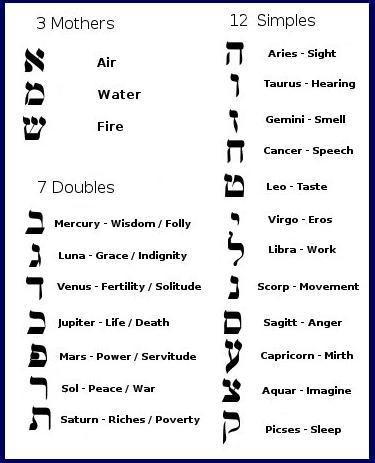The names of the five planets, one star and one moon [planetary satellite] are:

Shabbatai שבתאי, Saturn
Meaning: "the restful one," whose name is parallel to that of the Sabbath Day; the Seventh day; the day of rest. Esoterically, Saturn embodies Time itself. In the midst of time's passage, Saturn remains still and silent, drawing all endeavors to a close.
Tzedeq צדק, Jupiter
Meaning: "righteousness", as Jupiter is the embodiment of divine influx.
Meaning: "the red one"
Ḥammah חמה, the Sun
Meaning: "the hot one"
Kokhevet כוכבת, Nogah נוגה or Kokhav-Nogah כוכב נוגה, Venus
Meanings: "the she-planet," "the bright one," or "the bright planet," respectively
Kokhav כוכב, Mercury
Meaning: "the planet," since Mercury is the Principle of planetary influence, in and of itself. The Mercurial principle is that of multiplorancy. It embodies our means of adaptation, and represents the many facets of existence. In being deemed simply as "the planet", Mercury is presented as a blank slate; an open-ended modality of being.
Levanah לבנה, the Moon
Meaning: "the white one"

Planets of the Solar System: מערקור‎ (merkur), ווענוס‎ (venus), ערד‎ (erd), מאַרס‎ (mars), יופּיטער‎ (yupiter), סאַטורן‎ (saturn), אוראַנוס‎ (uranus), נעפּטון‎ (neptun)

Some of the planets perhaps were not known during the biblical era and do not have Hebrew names (Uranus and Neptune).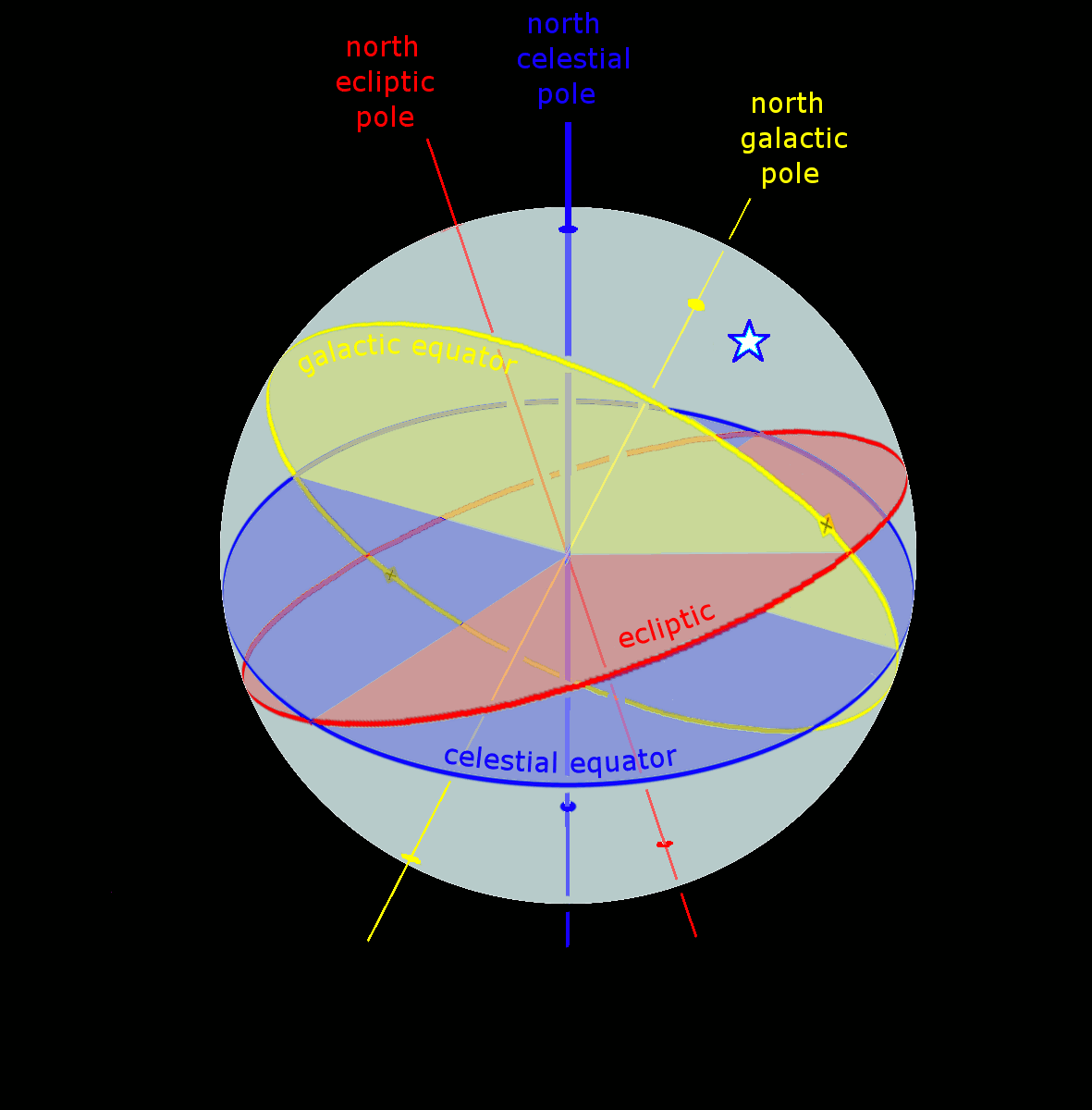Supergalactic coordinates are coordinates in a spherical coordinate system which was designed to have its equator aligned with the supergalactic plane, a major structure in the local universe formed by the preferential distribution of nearby galaxy clusters (such as the Virgo cluster, the Great Attractor and the Pisces-Perseus supercluster) towards a (two-dimensional) plane

The north supergalactic pole (SGB = 90°) lies at galactic coordinates (lz = 47.37°, bz = +6.32°). In the equatorial coordinate system (epoch J2000), this is approximately RA = 18.9h, Dec = +15.7°.
The zero point (SGB = 0°, SGL = 0°) lies at (lx = 137.37°, bx = 0°). In J2000 equatorial coordinates, this is approximately 2.82h, +59.5°. So the transformation from a triple of Cartesian supergalactic coordinates to a triple of galactic coordinates.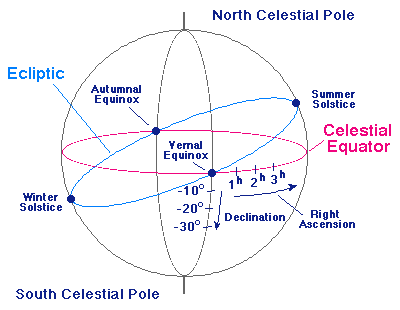Celestial coordinate system (or celestial reference system) is a system for specifying positions of satellites, planets, stars, galaxies, and other celestial objects. Coordinate systems can specify an object's position in three-dimensional space or plot merely its direction on a celestial sphere, if the object's distance is unknown or trivial.

A star's  galactic, ecliptic, and equatorial coordinates, as projected on the celestial sphere. Ecliptic and equatorial coordinates share the      March equinox as the primary direction, and galactic coordinates are referred to the      galactic center.

Earth-centered inertial (ECI) coordinate frames have their origins at the center of mass of Earth and do not rotate with respect to the stars. ECI frames are called inertial, in contrast to the Earth-centered, Earth-fixed (ECEF) frames, which remain fixed with respect to Earth's surface in its rotation. It is convenient to represent the positions and velocities of terrestrial objects in ECEF coordinates or with latitude, longitude, and altitude. However, for objects in space, the equations of motion that describe orbital motion are simpler in a non-rotating frame such as ECI. The ECI frame is also useful for specifying the direction toward celestial objects.

If the observable universe encompasses the entire universe, we may be able to determine the structure of the entire universe by observation. However, if the observable universe is smaller than the entire universe, our observations will be limited to only a part of the whole, and we may not be able to determine its global geometry through measurement
the data are also consistent with other possible shapes, such as the so-called Poincaré dodecahedral space and the Sokolov–Starobinskii space (quotient of the upper half-space model of hyperbolic space by 2-dimensional lattice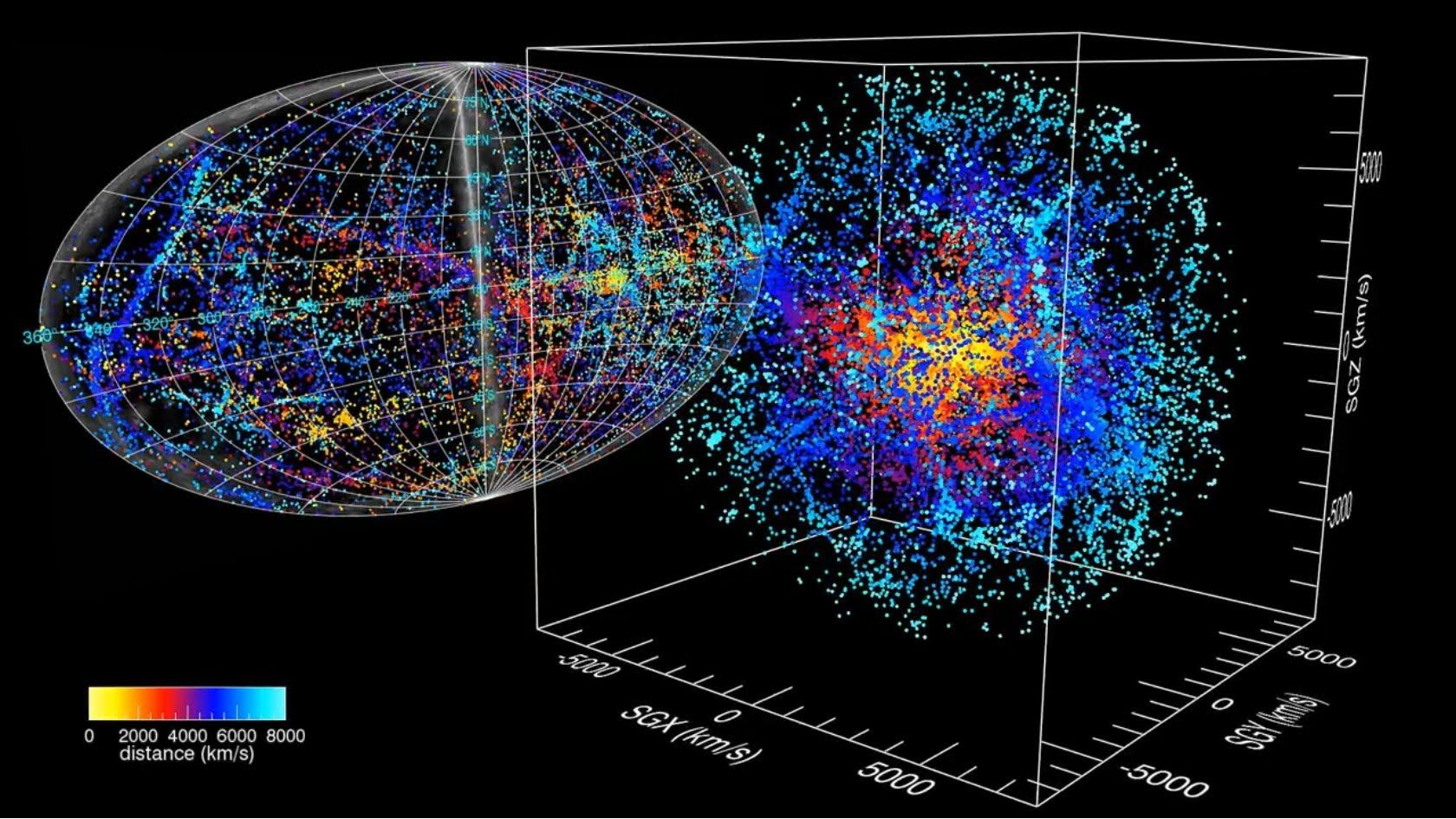The local geometry of the universe is determined by whether the density parameter Ω is greater than, less than, or equal to 1. A spherical universe with Ω > 1, a hyperbolic universe with Ω

Cyclosilicates (Ring Silicates)
If two of the oxygens are shared and the structure is arranged in a ring, the basic structural unit of the cyclosilcates or ring silicates.  Shown here is a six membered ring forming the structural group Si6O18-12.  Three membered rings, Si3O9-6, four membered rings, Si4O12-8, and five membered rings Si5O15-10 are also possible.  A good example of a cyclosilicate is the mineral Beryl - Be3Al2Si6O18.Nanoscalemetrology Soft Matter Dynamics
A Quasicrystal or quasi-periodic crystall is matter formed atomically in a manner somewhere between the amorphous solids of glasses (special forms of metals and other minerals, as well as common glass) and the precise pattern of crystals. Like crystals, they contain an ordered structure, but the patterns are subtle and do not recur at precisely regular intervals. They appear to be formed from two different structures assembled in a nonrepeating array, the three-dimensional equivalent of a tile floor made from two shapes of tile and having an orientational order but no repetition. Although when first discovered such structures surprised the scientific community, it now appears that quasicrystals rank among the most common structures in alloys of aluminum with such metals as iron, cobalt, or nickel. While no major commercial applications yet exploit properties of the quasicrystalline state directly, quasicrystals form in compounds noted for their high strength and light weight, suggesting potential applications in aerospace and other industries.Electron microscope and quasicrystals?
It is a versatile tool that can probe many important aspects of the structure of matter. Low-resolution scanning electron microscopy magnifies the shapes of individual grains. Symmetries of solid grains often reflect the internal symmetries of the underlying atomic positions. Grains of salt, for example, take cubical shapes consistent with the cubic symmetries of their crystal lattices. Quasicrystalline aluminum-copper-iron has been imaged using a scanning electron microscope, revealing the pentagonal dodecahedral shape of the grains. Its 12 faces are regular pentagons, with axes of fivefold rotational symmetry passing through them. That is to say, rotations about this axis by 72° leave the appearance of the grain unchanged. In a full 360° rotation the grain will repeat itself in appearance five times, once every 72°. There are also axes of twofold rotational symmetry passing through the edges and axes of threefold rotational symmetry passing through the vertices. This is also known as icosahedral symmetry because the icosahedron is the geometric dual of the pentagonal dodecahedron. At the centre of each face on an icosahedron, the dodecahedron places a vertex, and vice versa. The symmetry of a pentagonal dodecahedron or icosahedron is not among the symmetries of any crystal structure, yet this is the symmetry that was revealed in the electron microscope image of the aluminum-manganese alloy .Fivefold symmetry axes are forbidden in ordinary crystals, while other axes, such as sixfold axes, are allowed. The reason is that translational periodicity, which is characteristic of crystal lattices, cannot be present in structures with fivefold symmetry. High-resolution electron microscopy magnifies to such a great degree that patterns of atomic positions may be determined. In ordinary crystals such a lattice image reveals regularly spaced rows of atoms. Regular spacing implies spatial periodicity in the placement of atoms. The angles between rows indicate rotational symmetries of the atomic positions. In a high-resolution electron microscope image of quasicrystalline aluminum-manganese-silicon, parallel rows occur in five sets, rotated from one another by 72°, confirming that the fivefold symmetry suggested by the shape of the pentagonal dodecahedron grain reflects a fivefold symmetry in the actual placement of atoms.

Translational periodicity and symmetry in the fivefold symmetry axes are forbidden in ordinary crystals, while other axes, such as sixfold axes, are allowed. Assume they are the shortest such displacements consider the combinations of two symmetries a and b with the net result c. The length of c is smaller than either a or b by a factor τ = (Square root of√5 + 1)/2, which is known as the golden mean. The new atomic position, outlined with a dotted line does not coincide with a previous atomic position, indicating that the structure does not exhibit translational periodicity. An array of atoms may not simultaneously display fivefold rotational symmetry and translational periodicity, if it did there would be no lower limit to the spacing between atoms.Density functional theory with the jellium model show that clusters with triangular [in two dimensions (2D)] or tetrahedral [in three dimensions (3D)] shapes have a strong shell structure and enhanced stability. The shell closings correspond to the lowest magic numbers of a 2D and 3D harmonic oscillator and at the same time to the number of divalent atoms in close-packed triangles and tetrahedrons. The Molecular dynamics and simulations clusters support the results of the jellium model. Asymptotic numbers of multidimensional partitions of an integer and directed as compact female god that formed dna crypto 64-lattice quantum spirit animals is the obvious explanation for evolution. "J"Gods First Number
Fifty-three is the 16th prime number. It is also an Eisenstein prime, and a Sophie Germain prime.
The sum of the first 53 primes is 5830, which is divisible by 53, a property shared by few other numbers.
53 written in hexadecimal is 35, that is, the same characters used in the decimal representation, but reversed. Four additional multiples of 53 share this property: 371 = 17316, 5141 = 141516, 99481 = 1849916, and 8520280 = 082025816. Apart from the trivial case of single-digit decimals, no other number has this property.
53 cannot be expressed as the sum of any integer and its base-10 digits, making 53 a self number.
53 is the smallest prime number that does not divide the order of any sporadic group

https://goldvoice.club/steem/@j1337/the-bible-triangle-prophecy-code-of-gematria-t-numbers

https://goldvoice.club/steem/@j1337/holy-pi-the-future-tesla-coil-free-energy-and-healing-searching-for-et-and-control-part-1

https://goldvoice.club/steem/@j1337/holy-pi-trinity-da-vinci-archimedes-puzzle-devils-pi-sphinx-riddle-solved-solomon-s-temple-part-2

https://goldvoice.club/steem/@j1337/solving-the-rubix-cube-s-with-god-s-number-s-and-the-devil-s-algorithm-s-777

https://goldvoice.club/steem/@j1337/733-337-top-secret-confidential-for-your-eyes-only-73-37

https://goldvoice.club/steem/@j1337/meta-mathematics-perfect-cube-uni-matrix-star-theory-sirisys-and-friends

06.11.2019 08:11
1